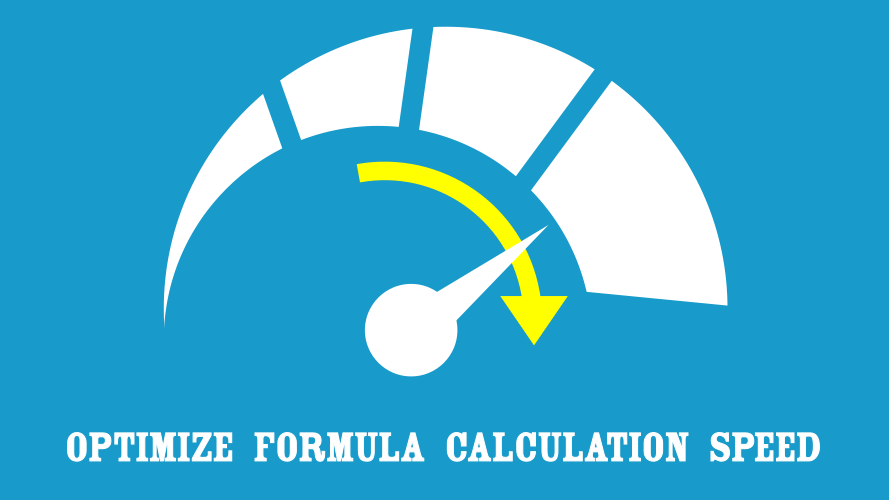# Optimize Formula Calculation Speed – Part 3: Volatile formulas## Part 3: Volatile formulas

Today we are going to look at volatile formulas.  This is the slightly odd description for a group of formulas that recalculate even if there are no changes to recalculate.

Excel is pretty clever, it keeps a track of all formulas and how they all interrelate.  For example: cell A3 references cell A2, and cell A2 references a value in cell A1.  Excel knows that it must calculate cell A2, then cell A3.  On the first calculation of the workbook it will calculate both cells. On the second calculation, Excel does not recalculate anything as nothing has changed in those cells.  This clever calculation process ensures that Excel does not need to re-calculate everything every time.  As result, calculation times are significantly reduced.

However, if cell A2 were a volatile formula then cells A2 and A3 would be recalculated every single time.  If the formula had exactly the same result as before, it will still recalculate cells A2 and A3.  This results in much slower recalculation times.  It is important to understand volatile formulas, as they are significant when trying to optimize formula calculation speed.

The key point to remember with volatile formulas is this: every cell that is in any way dependent on a volatile formula is recalculated every time!  If you have a worksheet where every cell is dependent on a cell containing a volatile formula, then every cell will be recalculated every time!  This could be a very big waste of your time.

## What triggers volatile formulas to recalculate?

Unfortunately, it is not just the act of recalculation which causes volatile formulas to recalculate.  The following will also cause the recalculation of volatile formulas:

• Renaming a worksheet
• Creation, editing or deleted a named range
• Moving the position of a worksheet tab
• Inserting or deleting rows and columns
• Using auto filter (though not on every action)
• Hiding or unhiding rows (though hiding and unhiding columns does not trigger a recalculation)
• Entering data (if in automatic calculation mode)

## Which formulas are the volatile?

This list may not be exhaustive, but the volatile formulas which I’m aware of are

## Alternatives to volatile formulas

The good news is that there are alternatives to most volatile formulas.

#### OFFSET

In most circumstances we can substitute the OFFSET function with the INDEX function.  There is a subtle difference between the two.  INDEX requires the first argument to be the complete range of cells in which it looks for the results, with the first cell in that range being the start cell.  However, OFFSET does not care about the full range of cells, it only cares about where the single start cell is.

#### INDIRECT

There is not a like-for-like replacement for the INDIRECT function. However, often there are ways to avoid needing to use INDIRECT within a worksheet.  By using column numbers, rather than letters it is possible to use the INDEX function to reference another cell.  If we are trying to reference across worksheets or workbooks there is not an alternative.

#### NOW & TODAY

There is no direct alternative to NOW or TODAY.  In most circumstances hard coding the value would be a suitable option.  However, if that is not possible, then try to minimise the number of cells which are dependent on the NOW and TODAY formulas.

Writing a VBA procedure to run whenever a workbook open is opened may be a good workaround.

For the NOW function we could use:

```Private Sub Workbook_Open()
Range("A1").Value =  Time
End Sub```

For the TODAY function we could use:

```Private Sub Workbook_Open()
Range("A1").Value = Date
End Sub```

#### RAND, RANDBETWEEN & RANDARRAY

How often do we need a new random number after each recalculation?  Almost never.  Which means the RAND, RANDBETWEEN and RANDARRAY functions are probably not required most of the time.  In most circumstances hard coding the value would be a suitable option.  However, if we do require a new random number we could use a VBA function to generate it based on the click of a button on the opening of the workbook.

To have random value created on workbook open we could use the following VBA code:

```Private Sub Workbook_Open()
Range("A1").Value = Rnd()
End Sub```

To have a RANDBETWEEN value recalculated on each workbook open we could use the same VBA above with the following formula in the worksheet.

=(HighValueLowValue) * RandomNumberCell + LowValue

#### INFO & CELL

INFO and CELL are not particularly common formulas.  They provide system, workbook and cell status information.  Some of this information is available in VBA (for example: the file path or cell color reference number).

## Are volatile formulas always bad?

No, volatile formulas are not always bad; they just need to be treated with a bit of care.  In a situation where we want every cell to re-calculate everything, then they are no worse than any other formulas.  But, for normal use, non-volatile alternatives are a better option.